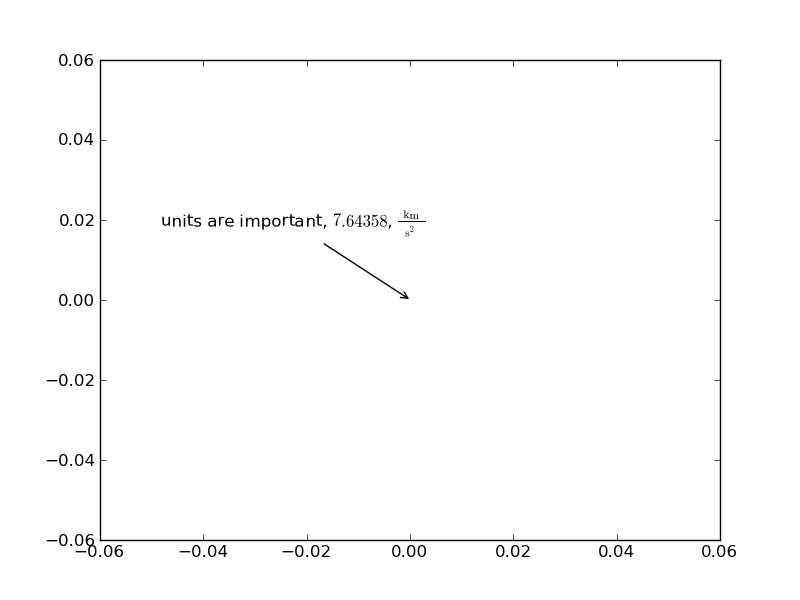# Text annotation misaligned with png backend.

Hello List,

I am so far happy with the output form matplotlib, but I recently started annotating my plots, and when saving to png-format, the annotations do not look very nice. When saving to eps format, I do not see the same problems.

Saving to eps and converting to png seems to be a good workaround for me.

The problem is that the letters in the same word (on the same line) are not aligned, some are drawn to far up or down.

png and eps figures are attached. Example script and version number (0.99.0) below.

Am I misusing matplotlib? Is this a bug?

Regards

johan

Minimal example:

pyplot_text.eps (29.2 KB)···

-----------------------
import matplotlib.pyplot as plt
import numpy as np

plt.figure()

x = 
plt.plot(x, x)

plt.annotate("units are important, $7.64358$, $\\frac{\mathrm{k}\mathrm{m}}{\mathrm{s^2}}$",
xy = (0,0),
xycoords = "data",
xytext = (0.2, 0.6),
textcoords = "figure fraction",
arrowprops = dict(arrowstyle = "->",
connectionstyle = "arc"))

plt.savefig("pyplot_text.png")
plt.savefig("pyplot_text.eps")
plt.show()
-----------------------

Expected result: An empty plot with a nice annotation on screen, in a png-file, and in an eps-file

Result: Empty plots with annotation displaying differently in the three cases. In the png file, the letters u and r and the digit 7 appear slightly above the correct position, and the m in the unit km appears slightly below the correct position. In the screen output, the m is correct, but the u and r show the same problem as in the png file. In the eps file, all seems to be well.

My os is debian (testing), and I obtain matplotlib and all related software from the debian repositories.

johan@...2848...:~$uname -a Linux johan-laptop 2.6.30-2-amd64 #1 SMP Fri Sep 25 22:16:56 UTC 2009 x86_64 GNU/Linux johan@...2848...:~$ python -c "import matplotlib; print matplotlib.__version__"
0.99.0

I don't see such problem in my installations.
I may be wrong but It seems to me that it is a font issue (I believe
you're not using usetex mode).

Can you try to use other fonts?

For example, my current installation picks up the following font (Vera Serif?).

In : matplotlib.font_manager.findfont(None)
Out: '/astro/research/jul27/local/lib/python2.5/site-packages/matplotlib/mpl-data/fonts/ttf/VeraSe.ttf'

Regards,

-JJ

2009/10/30 Johan Grönqvist <johan.gronqvist@...287...>:

···

Hello List,

I am so far happy with the output form matplotlib, but I recently started
annotating my plots, and when saving to png-format, the annotations do not
look very nice. When saving to eps format, I do not see the same problems.

Saving to eps and converting to png seems to be a good workaround for me.

The problem is that the letters in the same word (on the same line) are not
aligned, some are drawn to far up or down.

png and eps figures are attached. Example script and version number (0.99.0)
below.

Am I misusing matplotlib? Is this a bug?

Regards

johan

Minimal example:
-----------------------
import matplotlib.pyplot as plt
import numpy as np

plt.figure()

x = 
plt.plot(x, x)

plt.annotate("units are important, $7.64358$,
$\\frac{\mathrm{k}\mathrm{m}}{\mathrm{s^2}}$",
xy = (0,0),
xycoords = "data",
xytext = (0.2, 0.6),
textcoords = "figure fraction",
arrowprops = dict(arrowstyle = "->",
connectionstyle = "arc"))

plt.savefig("pyplot_text.png")
plt.savefig("pyplot_text.eps")
plt.show()
-----------------------

Expected result: An empty plot with a nice annotation on screen, in a
png-file, and in an eps-file

Result: Empty plots with annotation displaying differently in the three
cases. In the png file, the letters u and r and the digit 7 appear slightly
above the correct position, and the m in the unit km appears slightly below
the correct position. In the screen output, the m is correct, but the u and
r show the same problem as in the png file. In the eps file, all seems to be
well.

My os is debian (testing), and I obtain matplotlib and all related software
from the debian repositories.

johan@...2848...:~$uname -a Linux johan-laptop 2.6.30-2-amd64 #1 SMP Fri Sep 25 22:16:56 UTC 2009 x86_64 GNU/Linux johan@...2848...:~$ python -c "import matplotlib; print
matplotlib.__version__"
0.99.0

------------------------------------------------------------------------------
Come build with us! The BlackBerry(R) Developer Conference in SF, CA
is the only developer event you need to attend this year. Jumpstart your
developing skills, take BlackBerry mobile applications to market and stay